Anúncio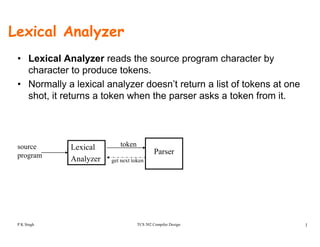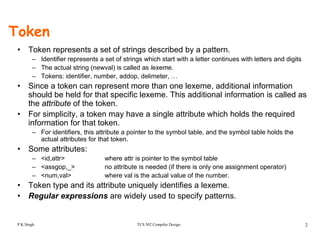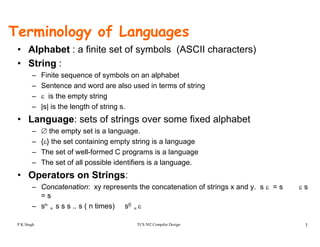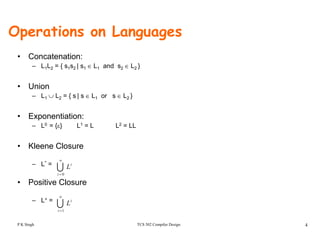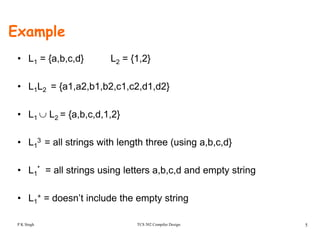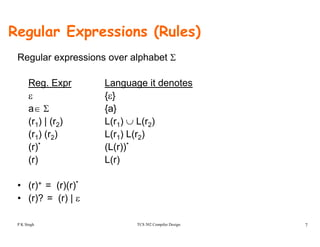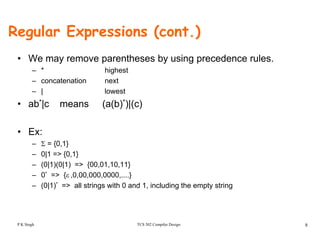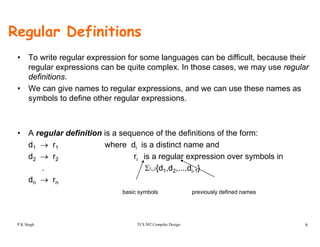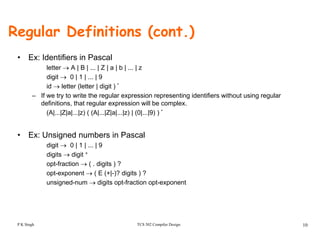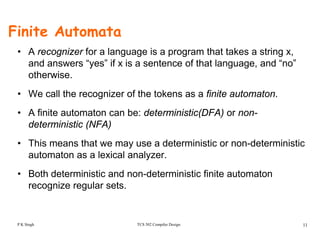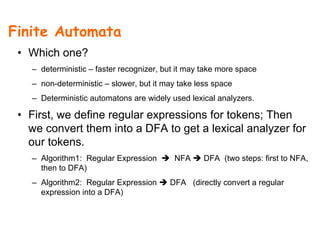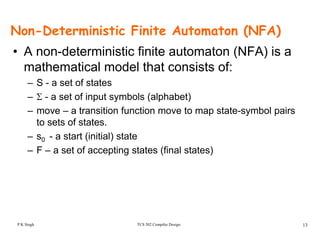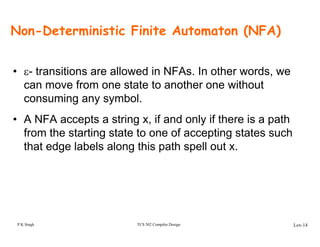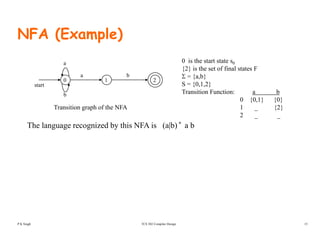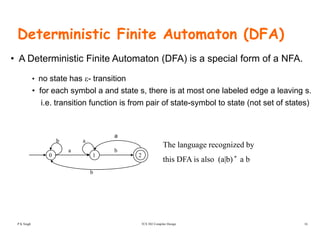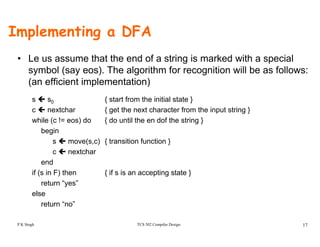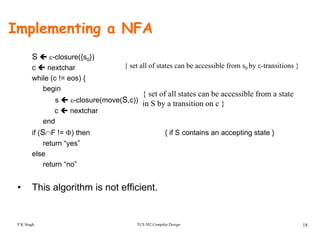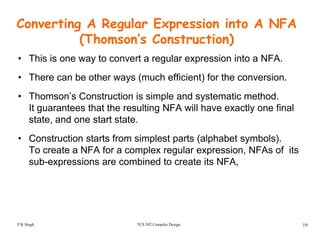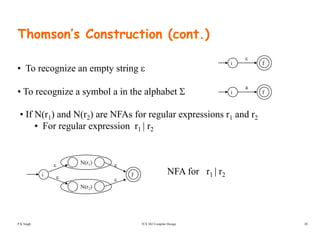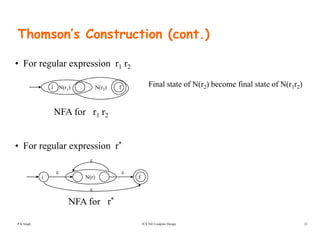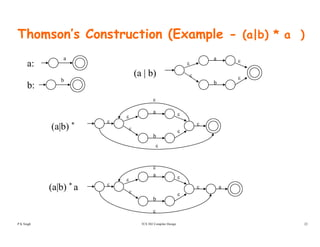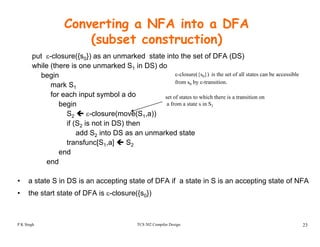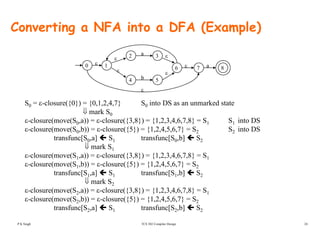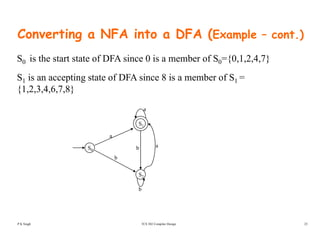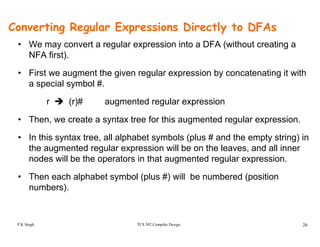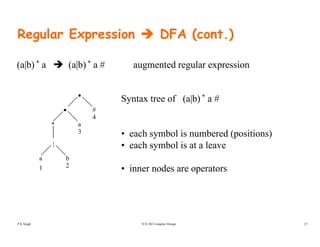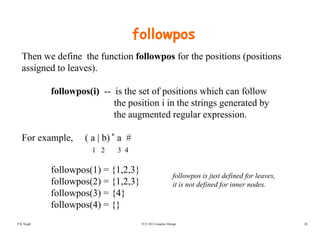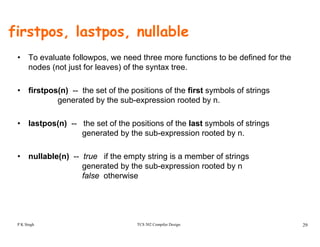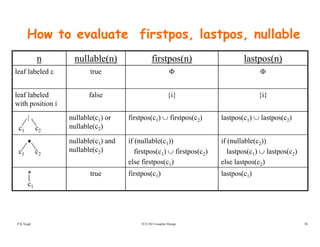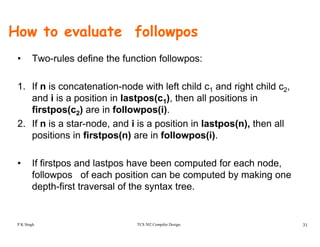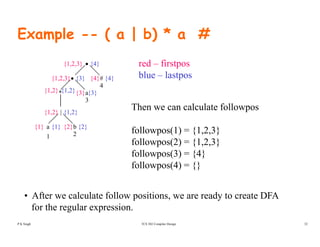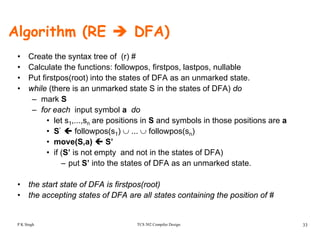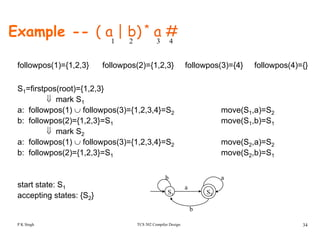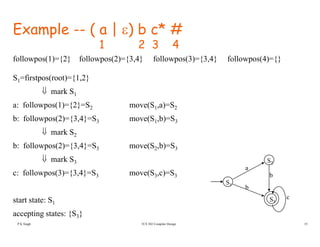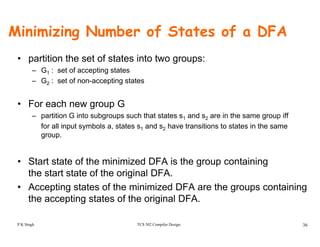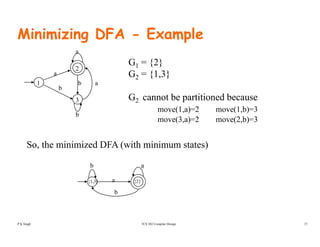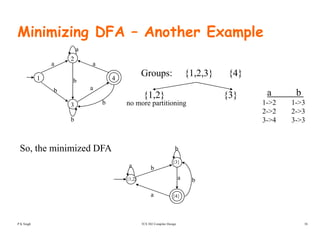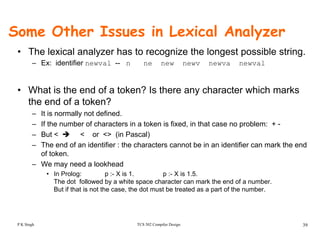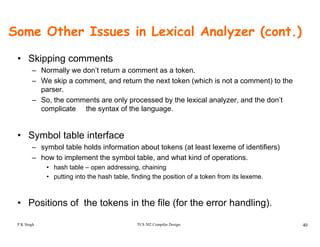1 de 40
Anúncio

### Lexicalanalyzer

1. Lexical Analyzer • Lexical Analyzer reads the source program character by character to produce tokens. • Normally a lexical analyzer doesn’t return a list of tokens at one shot, it returns a token when the parser asks a token from it. Lexical Analyzer Parser source program token get next token TCS 502 Compiler Design 1P K Singh
2. Token • Token represents a set of strings described by a pattern. – Identifier represents a set of strings which start with a letter continues with letters and digits – The actual string (newval) is called as lexeme. – Tokens: identifier number addop delimeter– Tokens: identifier, number, addop, delimeter, … • Since a token can represent more than one lexeme, additional information should be held for that specific lexeme. This additional information is called as the attribute of the token. • For simplicity, a token may have a single attribute which holds the required information for that token. – For identifiers, this attribute a pointer to the symbol table, and the symbol table holds the actual attributes for that tokenactual attributes for that token. • Some attributes: – <id,attr> where attr is pointer to the symbol table – <assgop,_> no attribute is needed (if there is only one assignment operator)g p _ ( y g p ) – <num,val> where val is the actual value of the number. • Token type and its attribute uniquely identifies a lexeme. • Regular expressions are widely used to specify patterns. TCS 502 Compiler Design 2P K Singh
3. Terminology of Languages f f ( SC )• Alphabet : a finite set of symbols (ASCII characters) • String : – Finite sequence of symbols on an alphabetFinite sequence of symbols on an alphabet – Sentence and word are also used in terms of string – ε is the empty string – |s| is the length of string s|s| is the length of string s. • Language: sets of strings over some fixed alphabet – ∅ the empty set is a language. { } th t t i i t t i i l– {ε} the set containing empty string is a language – The set of well-formed C programs is a language – The set of all possible identifiers is a language. • Operators on Strings: – Concatenation: xy represents the concatenation of strings x and y. s ε = s ε s = s TCS 502 Compiler Design 3 – sn = s s s .. s ( n times) s0 = ε P K Singh
4. Operations on Languages • Concatenation: – L1L2 = { s1s2 | s1 ∈ L1 and s2 ∈ L2 } • Union – L1 ∪ L2 = { s | s ∈ L1 or s ∈ L2 } • Exponentiation: – L0 = {ε} L1 = L L2 = LL • Kleene Closure – L* = U ∞ i L • Positive Closure – L+ = U= 0i U ∞ i L TCS 502 Compiler Design 4 L U=1i i L P K Singh
5. Example • L1 = {a,b,c,d} L2 = {1,2} • L1L2 = {a1,a2,b1,b2,c1,c2,d1,d2} • L1 ∪ L2 = {a,b,c,d,1,2} L 3 ll t i ith l th th ( i b d}• L1 3 = all strings with length three (using a,b,c,d} • L * = all strings using letters a b c d and empty string• L1 = all strings using letters a,b,c,d and empty string • L1 + = doesn’t include the empty string TCS 502 Compiler Design 5 1 does t c ude t e e pty st g P K Singh
6. Regular Expressions • We use regular expressions to describe tokens of a programming language. • A regular expression is built up of simpler regular expressions (using defining rules) Each regular expression denotes a language• Each regular expression denotes a language. • A language denoted by a regular expression is called as a regular set.g TCS 502 Compiler Design 6P K Singh
7. Regular Expressions (Rules) Regular expressions over alphabet Σ Reg. Expr Language it denotes ε {ε} a∈ Σ {a}a∈ Σ {a} (r1) | (r2) L(r1) ∪ L(r2) (r1) (r2) L(r1) L(r2)( 1) ( 2) ( 1) ( 2) (r)* (L(r))* (r) L(r) • (r)+ = (r)(r)* • (r)? = (r) | ε TCS 502 Compiler Design 7 ( ) ( ) | ε P K Singh
8. Regular Expressions (cont.) • We may remove parentheses by using precedence rules. – * highest – concatenation next – | lowest • ab*|c means (a(b)*)|(c)| ( ( ) )|( ) • Ex: – Σ = {0,1} – 0|1 => {0,1} – (0|1)(0|1) => {00,01,10,11} – 0* => {ε ,0,00,000,0000,....} – (0|1)* => all strings with 0 and 1, including the empty string TCS 502 Compiler Design 8P K Singh
9. Regular Definitions • To write regular expression for some languages can be difficult, because their regular expressions can be quite complex. In those cases, we may use regular definitionsdefinitions. • We can give names to regular expressions, and we can use these names as symbols to define other regular expressions. • A regular definition is a sequence of the definitions of the form: d1 → r1 where di is a distinct name and d2 → r2 ri is a regular expression over symbols in . Σ∪{d1,d2,...,di 1}. Σ∪{d1,d2,...,di-1} dn → rn basic symbols previously defined names TCS 502 Compiler Design 9P K Singh
10. Regular Definitions (cont.) • Ex: Identifiers in Pascal letter → A | B | ... | Z | a | b | ... | z digit → 0 | 1 | | 9digit → 0 | 1 | ... | 9 id → letter (letter | digit ) * – If we try to write the regular expression representing identifiers without using regular definitions, that regular expression will be complex. (A|...|Z|a|...|z) ( (A|...|Z|a|...|z) | (0|...|9) ) * • Ex: Unsigned numbers in Pascalg digit → 0 | 1 | ... | 9 digits → digit + opt-fraction → ( . digits ) ? opt-exponent → ( E (+|-)? digits ) ? unsigned-num → digits opt-fraction opt-exponent TCS 502 Compiler Design 10P K Singh
11. Finite Automata • A recognizer for a language is a program that takes a string x, and answers “yes” if x is a sentence of that language, and “no” otherwiseotherwise. • We call the recognizer of the tokens as a finite automaton. • A finite automaton can be: deterministic(DFA) or non- deterministic (NFA) • This means that we may use a deterministic or non-deterministic automaton as a lexical analyzer. B h d i i i d d i i i fi i• Both deterministic and non-deterministic finite automaton recognize regular sets. TCS 502 Compiler Design 11P K Singh
12. Finite Automata • Which one? – deterministic – faster recognizer, but it may take more space – non-deterministic – slower, but it may take less space – Deterministic automatons are widely used lexical analyzers. • First, we define regular expressions for tokens; Then we convert them into a DFA to get a lexical analyzer for t kour tokens. – Algorithm1: Regular Expression NFA DFA (two steps: first to NFA, then to DFA)then to DFA) – Algorithm2: Regular Expression DFA (directly convert a regular expression into a DFA)
13. Non-Deterministic Finite Automaton (NFA) • A non-deterministic finite automaton (NFA) is a mathematical model that consists of: – S - a set of states – Σ - a set of input symbols (alphabet) t iti f ti t t t b l i– move – a transition function move to map state-symbol pairs to sets of states. – s0 - a start (initial) states0 a start (initial) state – F – a set of accepting states (final states) TCS 502 Compiler Design 13P K Singh
14. Non-Deterministic Finite Automaton (NFA) • ε- transitions are allowed in NFAs. In other words, we can move from one state to another one without consuming any symbol. • A NFA accepts a string x, if and only if there is a path from the starting state to one of accepting states such that edge labels along this path spell out x. P K Singh TCS 502 Compiler Design Lex-14
15. NFA (Example) a b a 0 is the start state s0 {2} is the set of final states F Σ = {a b} 10 2 a b start b Σ = {a,b} S = {0,1,2} Transition Function: a b 0 {0,1} {0} 1 {2}Transition graph of the NFA 1 _ {2} 2 _ _ Transition graph of the NFA The language recognized by this NFA is (a|b) * a b TCS 502 Compiler Design 15P K Singh
16. Deterministic Finite Automaton (DFA) • A Deterministic Finite Automaton (DFA) is a special form of a NFA. t t h t iti• no state has ε- transition • for each symbol a and state s, there is at most one labeled edge a leaving s. i.e. transition function is from pair of state-symbol to state (not set of states) a b 10 2 ba b The language recognized by this DFA is also (a|b) * a b b a b TCS 502 Compiler Design 16P K Singh
17. Implementing a DFA • Le us assume that the end of a string is marked with a special symbol (say eos). The algorithm for recognition will be as follows: (an efficient implementation) s s0 { start from the initial state } t h { t th t h t f th i t t i }c nextchar { get the next character from the input string } while (c != eos) do { do until the en dof the string } begin ( ) { t iti f ti }s move(s,c) { transition function } c nextchar end if ( i F) th { if i ti t t }if (s in F) then { if s is an accepting state } return “yes” else “ ” TCS 502 Compiler Design 17 return “no” P K Singh
18. Implementing a NFA S ε-closure({s0}) c nextchar hile (c ! eos) { { set all of states can be accessible from s0 by ε-transitions } while (c != eos) { begin s ε-closure(move(S,c)) { set of all states can be accessible from a state in S by a transition on c } c nextchar end if (S∩F != Φ) then { if S contains an accepting state } return “yes” else return “no” • This algorithm is not efficient. TCS 502 Compiler Design 18P K Singh
19. Converting A Regular Expression into A NFA (Thomson’s Construction)( ) • This is one way to convert a regular expression into a NFA. • There can be other ways (much efficient) for the conversion• There can be other ways (much efficient) for the conversion. • Thomson’s Construction is simple and systematic method. It guarantees that the resulting NFA will have exactly one finalIt guarantees that the resulting NFA will have exactly one final state, and one start state. • Construction starts from simplest parts (alphabet symbols)• Construction starts from simplest parts (alphabet symbols). To create a NFA for a complex regular expression, NFAs of its sub-expressions are combined to create its NFA, TCS 502 Compiler Design 19P K Singh
20. Thomson’s Construction (cont.) • To recognize an empty string ε fi ε • To recognize a symbol a in the alphabet Σ a fi • If N(r1) and N(r2) are NFAs for regular expressions r1 and r2 • For regular expression r1 | r2 N(r1) NFA for r | r ε ε N(r2) fi NFA for r1 | r2 εε TCS 502 Compiler Design 20P K Singh
21. Thomson’s Construction (cont.) • For regular expression r1 r2 i fN(r2)N(r1) Final state of N(r2) become final state of N(r1r2) NFA for r1 r2 • For regular expression r* ε N(r)i f NFA for r* ε ε ε TCS 502 Compiler Design 21 NFA for r P K Singh
22. Thomson’s Construction (Example - (a|b) * a ) a: a (a | b) a ε ε ε b b: ( | ) b εε ε b ε ε ε ε a ε ε (a|b) * ε ε b ε ε ε ε a ε ε a(a|b) * a TCS 502 Compiler Design 22 ε P K Singh
23. Converting a NFA into a DFA (subset construction)( ) put ε-closure({s0}) as an unmarked state into the set of DFA (DS) while (there is one unmarked S1 in DS) do begin ε-closure({s0}) is the set of all states can be accessiblebegin mark S1 for each input symbol a do begin set of states to which there is a transition on a from a state s in S1 ({ 0}) from s0 by ε-transition. g S2 ε-closure(move(S1,a)) if (S2 is not in DS) then add S2 into DS as an unmarked state transfunc[S1,a] S2 end end • a state S in DS is an accepting state of DFA if a state in S is an accepting state of NFA • the start state of DFA is ε-closure({s0}) TCS 502 Compiler Design 23P K Singh
24. Converting a NFA into a DFA (Example) b ε ε ε ε a ε ε a0 1 32 7 86 b ε 4 5 S0 = ε-closure({0}) = {0,1,2,4,7} S0 into DS as an unmarked state ⇓⇓ mark S0 ε-closure(move(S0,a)) = ε-closure({3,8}) = {1,2,3,4,6,7,8} = S1 S1 into DS ε-closure(move(S0,b)) = ε-closure({5}) = {1,2,4,5,6,7} = S2 S2 into DS transfunc[S0 a] S1 transfunc[S0 b] S2transfunc[S0,a] S1 transfunc[S0,b] S2 ⇓ mark S1 ε-closure(move(S1,a)) = ε-closure({3,8}) = {1,2,3,4,6,7,8} = S1 ε-closure(move(S1,b)) = ε-closure({5}) = {1,2,4,5,6,7} = S2 f f btransfunc[S1,a] S1 transfunc[S1,b] S2 ⇓ mark S2 ε-closure(move(S2,a)) = ε-closure({3,8}) = {1,2,3,4,6,7,8} = S1 ε-closure(move(S2,b)) = ε-closure({5}) = {1,2,4,5,6,7} = S2 TCS 502 Compiler Design 24 ε closure(move(S2,b)) ε closure({5}) {1,2,4,5,6,7} S2 transfunc[S2,a] S1 transfunc[S2,b] S2 P K Singh
25. Converting a NFA into a DFA (Example – cont.) S0 is the start state of DFA since 0 is a member of S0={0,1,2,4,7} S is an accepting state of DFA since 8 is a member of S =S1 is an accepting state of DFA since 8 is a member of S1 = {1,2,3,4,6,7,8} a a S1 b abS0 b S2 TCS 502 Compiler Design 25P K Singh
26. Converting Regular Expressions Directly to DFAs • We may convert a regular expression into a DFA (without creating aWe may convert a regular expression into a DFA (without creating a NFA first). • First we augment the given regular expression by concatenating it withg g g p y g a special symbol #. r (r)# augmented regular expression • Then, we create a syntax tree for this augmented regular expression. • In this syntax tree all alphabet symbols (plus # and the empty string) inIn this syntax tree, all alphabet symbols (plus # and the empty string) in the augmented regular expression will be on the leaves, and all inner nodes will be the operators in that augmented regular expression. • Then each alphabet symbol (plus #) will be numbered (position numbers). TCS 502 Compiler Design 26P K Singh
27. Regular Expression DFA (cont.) (a|b) * a (a|b) * a # augmented regular expression • # Syntax tree of (a|b) * a # * • a # 4 3 • each symbol is numbered (positions) | ba 1 2 • each symbol is at a leave • inner nodes are operatorsinner nodes are operators TCS 502 Compiler Design 27P K Singh
28. followpos Then we define the function followpos for the positions (positions assigned to leaves). followpos(i) -- is the set of positions which can follow the position i in the strings generated byp g g y the augmented regular expression. For example ( a | b) * a #For example, ( a | b) a # 1 2 3 4 followpos(1) = {1 2 3}followpos(1) = {1,2,3} followpos(2) = {1,2,3} followpos(3) = {4} f ll (4) {} followpos is just defined for leaves, it is not defined for inner nodes. TCS 502 Compiler Design 28 followpos(4) = {} P K Singh
29. firstpos, lastpos, nullable • To evaluate followpos, we need three more functions to be defined for the nodes (not just for leaves) of the syntax tree. • firstpos(n) -- the set of the positions of the first symbols of strings generated by the sub-expression rooted by n. • lastpos(n) -- the set of the positions of the last symbols of strings generated by the sub-expression rooted by n. • nullable(n) -- true if the empty string is a member of strings generated by the sub-expression rooted by ng y p y false otherwise TCS 502 Compiler Design 29P K Singh
30. How to evaluate firstpos, lastpos, nullable n nullable(n) firstpos(n) lastpos(n) leaf labeled ε true Φ Φ leaf labeled with position i false {i} {i} | c1 c2 nullable(c1) or nullable(c2) firstpos(c1) ∪ firstpos(c2) lastpos(c1) ∪ lastpos(c2) ll bl ( ) d if ( ll bl ( )) if ( ll bl ( ))• c1 c2 nullable(c1) and nullable(c2) if (nullable(c1)) firstpos(c1) ∪ firstpos(c2) else firstpos(c1) if (nullable(c2)) lastpos(c1) ∪ lastpos(c2) else lastpos(c2) * c1 true firstpos(c1) lastpos(c1) TCS 502 Compiler Design 30P K Singh
31. How to evaluate followpos • Two-rules define the function followpos: 1. If n is concatenation-node with left child c1 and right child c2, and i is a position in lastpos(c1), then all positions in firstpos(c ) are in followpos(i)firstpos(c2) are in followpos(i). 2. If n is a star-node, and i is a position in lastpos(n), then all positions in firstpos(n) are in followpos(i).p p ( ) p ( ) • If firstpos and lastpos have been computed for each node, followpos of each position can be computed by making one depth-first traversal of the syntax tree. TCS 502 Compiler Design 31P K Singh
32. Example -- ( a | b) * a # • # {1,2,3} {1 2 3} {4} {4} {4}{3} red – firstpos blue – lastpos * • a # 4 3 {3} {1,2,3} {1,2} {4} {4}{3} {3}{1,2} blue lastpos Then we can calculate followpos| ba 1 2 {1}{1} {1,2} {2} {1,2} {2} Then we can calculate followpos followpos(1) = {1,2,3} f ll (2) {1 2 3}followpos(2) = {1,2,3} followpos(3) = {4} followpos(4) = {}p ( ) {} • After we calculate follow positions, we are ready to create DFA TCS 502 Compiler Design 32 for the regular expression. P K Singh
33. Algorithm (RE DFA) • Create the syntax tree of (r) # • Calculate the functions: followpos, firstpos, lastpos, nullable • Put firstpos(root) into the states of DFA as an unmarked state• Put firstpos(root) into the states of DFA as an unmarked state. • while (there is an unmarked state S in the states of DFA) do – mark S f h i t b l d– for each input symbol a do • let s1,...,sn are positions in S and symbols in those positions are a • S’ followpos(s1) ∪ ... ∪ followpos(sn) (S ) S’• move(S,a) S’ • if (S’ is not empty and not in the states of DFA) – put S’ into the states of DFA as an unmarked state. • the start state of DFA is firstpos(root) • the accepting states of DFA are all states containing the position of # TCS 502 Compiler Design 33P K Singh
34. Example -- ( a | b) * a #1 2 3 4 followpos(1)={1,2,3} followpos(2)={1,2,3} followpos(3)={4} followpos(4)={} S1=firstpos(root)={1,2,3} ⇓ mark S1 f ll (1) f ll (3) {1 2 3 4} S (S ) Sa: followpos(1) ∪ followpos(3)={1,2,3,4}=S2 move(S1,a)=S2 b: followpos(2)={1,2,3}=S1 move(S1,b)=S1 ⇓ mark S2 f ll (1) f ll (3) {1 2 3 4} S (S ) Sa: followpos(1) ∪ followpos(3)={1,2,3,4}=S2 move(S2,a)=S2 b: followpos(2)={1,2,3}=S1 move(S2,b)=S1 start state: S1 accepting states: {S2} S1 S2 a b a TCS 502 Compiler Design 34 b P K Singh
35. Example -- ( a | ε) b c* # 1 2 3 41 2 3 4 followpos(1)={2} followpos(2)={3,4} followpos(3)={3,4} followpos(4)={} S firstpos(root) {1 2}S1=firstpos(root)={1,2} ⇓ mark S1 a: followpos(1)={2}=S2 move(S1,a)=S2p ( ) { } 2 ( 1, ) 2 b: followpos(2)={3,4}=S3 move(S1,b)=S3 ⇓ mark S2 b: followpos(2)={3,4}=S3 move(S2,b)=S3 ⇓ mark S3 f ll (3) {3 4} S (S ) S S2 a c: followpos(3)={3,4}=S3 move(S3,c)=S3 start state: S1 S3 S1 c b b TCS 502 Compiler Design 35 start state: S1 accepting states: {S3} S3 P K Singh
36. Minimizing Number of States of a DFA • partition the set of states into two groups: – G1 : set of accepting states – G2 : set of non-accepting states • For each new group Gg p – partition G into subgroups such that states s1 and s2 are in the same group iff for all input symbols a, states s1 and s2 have transitions to states in the same group. • Start state of the minimized DFA is the group containing th t t t t f th i i l DFAthe start state of the original DFA. • Accepting states of the minimized DFA are the groups containing the accepting states of the original DFA. TCS 502 Compiler Design 36 the accepting states of the original DFA. P K Singh
37. Minimizing DFA - Example a a 2 G1 = {2} G2 = {1,3} b a b 3 1 G2 {1,3} G2 cannot be partitioned because (1 ) 2 (1 b) 3 b move(1,a)=2 move(1,b)=3 move(3,a)=2 move(2,b)=3 So, the minimized DFA (with minimum states) ab {1,3} a b {2} TCS 502 Compiler Design 37P K Singh
38. Minimizing DFA – Another Example a a a 4 2 1 Groups: {1,2,3} {4} b b a b 4 3 1 p { , , } { } a b 1->2 1->3 {1,2} {3} no more partitioning b 2->2 2->3 3->4 3->3 So, the minimized DFA {3} ba b {1,2} {4} a a b TCS 502 Compiler Design 38P K Singh
39. Some Other Issues in Lexical Analyzer • The lexical analyzer has to recognize the longest possible string. – Ex: identifier newval -- n ne new newv newva newval • What is the end of a token? Is there any character which marks the end of a token? – It is normally not defined. – If the number of characters in a token is fixed, in that case no problem: + - – But < < or <> (in Pascal) – The end of an identifier : the characters cannot be in an identifier can mark the end of token. – We may need a lookhead • In Prolog: p :- X is 1. p :- X is 1.5. The dot followed by a white space character can mark the end of a number. But if that is not the case, the dot must be treated as a part of the number. TCS 502 Compiler Design 39P K Singh
40. Some Other Issues in Lexical Analyzer (cont.) • Skipping comments – Normally we don’t return a comment as a token. – We skip a comment, and return the next token (which is not a comment) to the parser. – So, the comments are only processed by the lexical analyzer, and the don’t complicate the syntax of the languagecomplicate the syntax of the language. • Symbol table interfacey – symbol table holds information about tokens (at least lexeme of identifiers) – how to implement the symbol table, and what kind of operations. • hash table – open addressing, chainingp g, g • putting into the hash table, finding the position of a token from its lexeme. Positions of the tokens in the file (for the error handling) TCS 502 Compiler Design 40 • Positions of the tokens in the file (for the error handling). P K Singh
Anúncio